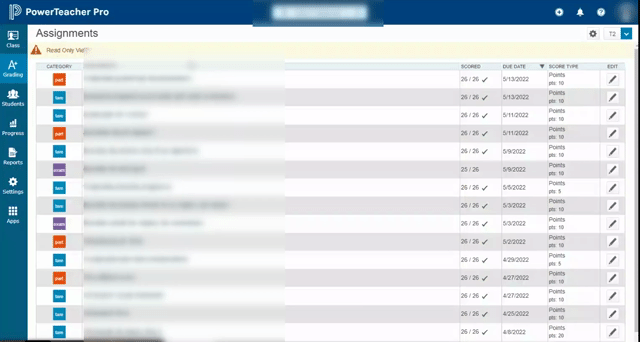Follow

# Grading: Exempting an Assignment or Final

Some circumstances call for excusing a student from an assignment, whether it's due to illness, personal circumstances or even because an assignment was optional. Read below to find out more about exempting an assignment.

### What does exempting an assignment do?

When a teacher exempts an assignment, whether it is a worksheet, quiz or final, PowerSchool will calculate the total grade as if the assignment does not exist for that student. The total point value in the category or class will drop to reflect the change. An exempt assignment is denoted with a purple slash mark, or, if a point value is entered, the point value will italicize and turn purple. Even if there is a point value for an exempt assignment, it will not count in the final grade total.

Here's an example: In Class A, students had to complete 5 homework assignments worth ten points each. Students 1 and 3 completed all of the assignments. Student 2 was exempted from one assignment, while Student 4 was exempted from two. See the total column for how this affects the point value of each students' grade.

 CLASS A HW 1 HW 2 HW 3 HW 4 HW 5 TOTAL Student 1 10 9 10 7 10 46/50 92% Student 2 9 10 10 / 8 37/40 92% Student 3 10 9 10 0 8 37/50 74% Student 4 10 / 5 10 10 30/30 100%

Note that Student 4 has an exempt assignment that is also graded, but those five points are not counted in the final grade.

Exempting an assignment has a powerful impact on a student's grade. Both Student 2 and Student 3 have the same point total, but while Student 2 was exempt from HW 4, Student 3 received a 0 for the assignment. That made a huge difference in their total grade.

### What does it mean to exempt a final?